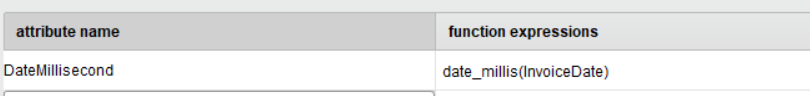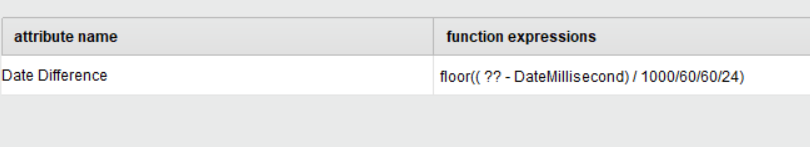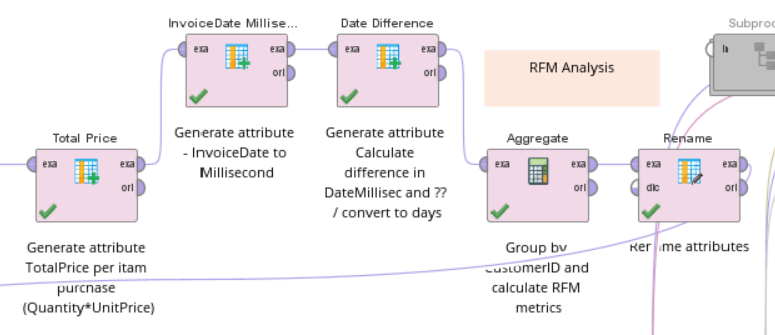# RFM scores - milliseconds

Member Posts: 1Newbie
Hello, i am trying to calculate RFM and i am stuck.

1)I saw that before the Aggregate operator (where calculate the R=max of InvoiceDate or min of InvoiceDateMillisecond, F=count of InvoiceNo, M=sum of TotalPrice) i need to calculate first the milliseconds from the InvoiceDate. Then should calculate also the difference between the milliseconds that i will create and another number again in millisecond, but i didn't understand which value will i use.
Also, can i find the Recency without the millisecond step?just to select "max InvoiceDate" in Aggregate operator?

2)How can i calculate RFM scores?

Any help?Thank youTagged: